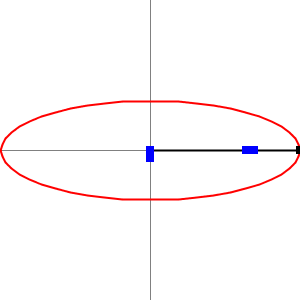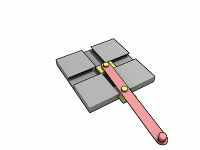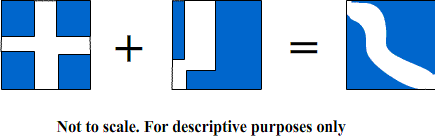_______
20110108---recorded on site 20110130

The “Trammel of Archimedes” or also known as “The Do Nothing Machine” is not a do nothing machine at all. It can be better described as a mechanical computer to plot orbits. As the two drawings from Wikipedia show, the machine creates an ellipse.

Where have we seen this before? Take a look at the “Extraordinary Ellipse.”

This machine can be used to determine vectors in statics or dynamics problems. The square (or can be another shape such as a rectangle) has a path that traces a vector. The shape of the path changes with different “circular” patterns. It is a path of the arms change as a radius does of the circle. The only difference is a circle has a fixed center. This center of the do nothing machine is moving.

As mentioned before the shape of the path can change. For more than 2 directions additional “squares” or patterns that occur at a different arm length can be used. Thus, there can be addition or subtraction between “squares.” For example to off-set squares can be added to form a curved path. This path would mean that the center of the “circle” drawing the orbit follows a curve instead of just a straight line path our “Trammel of Archimedes” does.

To find the “circumference” or length of the path around the ellipse by the change of radius or square lengths summated.

This is not right, but I propose something to the effect:
Summation from 0 to # radians of the [rate of change of radius * radius at given point]